[BACK]
 Computers, Materials & ContinuaDOI:10.32604/cmc.2021.018411Article

Modeling of Heart Rate Variability Using Time-Frequency Representations

1College of Engineering and Technology, American University of the Middle East, Kuwait
2Department of Mathematics and General Sciences, Prince Sultan University, Riyadh, 11586, Saudi Arabia
3Department of Medical Research, China Medical University, Taichung, 40402, Taiwan
4Department of Computer Science and Information Engineering, Asia University, Taichung, Taiwan
5Department of Electrical & Electronic Engineering, University of Turkish Aeronautical Association, Ankara, 06790, Turkey
Received: 06 March 2021; Accepted: 08 April 2021

Abstract: The heart rate variability signal is highly correlated with the respiration even at high workload exercise. It is also known that this phenomenon still exists during increasing exercise. In the current study, we managed to model this correlation during increasing exercise using the time varying integral pulse frequency modulation (TVIPFM) model that relates the mechanical modulation (MM) to the respiration and the cardiac rhythm. This modulation of the autonomic nervous system (ANS) is able to simultaneously decrease sympathetic and increase parasympathetic activity. The TVIPFM model takes into consideration the effect of the increasing exercise test, where the effect of a time-varying threshold on the heart period is studied. Our motivation is to analyze the heart rate variability (HRV) acquired by time varying integral pulse frequency modulation using time frequency representations. The estimated autonomic nervous system (ANS) modulating signal is filtered throughout the respiration using a time varying filtering, during exercise stress testing. And after summing power of the filtered signal, we compare the power of the filtered modulation of the ANS obtained with different time frequency representations: smoothed pseudo Wigner–Ville representation, spectrogram and their reassignments. After that, we used a student t-test p<0.01 to compare the power of heart rate variability in the frequency band of respiration and elsewhere.

Keywords: Heart rate variability; respiration; TVIPFM; mechanical modulation; autonomic nervous system

1  Introduction

The Heart Rate Variability is the time variation of heartbeats. It reflects the regulations of the Autonomic nervous System [1,2]. The fluctuations of heart rate are modeled by mathematical chaos . The variability leads to the flexibility to rapidly contend with a changing environment. Modeling biological systems reveals spatial and temporal complexity, so any disorder changes this complexity . Higher magnitude of the HRV is not always a sign of healthy biological conditions. For instance, any conduction problem in the heart, makes HRV amplitudes higher. To study the HRV, we need to start with studying electrocardiogram (ECG) waves. A good interpretation of these waves shows whether high HRV values are the consequence of cardiac problems like atrial fibrillation  or other side effects that could impact heart rhythm . A normal magnitude of HRV could be interpreted as a sign of adaptability or resilience. Higher magnitudes of HRV are due to the effect of executive functions by the prefrontal cortex [7,8].

The Integral pulse frequency modulation (IPFM) model explains the influence of the heart rate by the autonomic nervous system  in several physiological conditions: at rest or during exercise [10,11]. However, the IPFM model considers the heart period as a constant [12,13] which means that it considers a constant mean heart period . That’s why we use the time varying threshold IPFM (TVIPFM) model to analyze the heart rate variability during exercise. Applying this model leads to the approximation of a modulating signal taking into account the time varying threshold. Many studies highlighted the close relationship between respiration and HRV . That’s why in this paper, we analyze non-stationary (HRV) signals using time varying filtering based on the frequency band of respiration.

Our study is in stress testing exercise, permitting us to look the respiratory information to estimate parasympathetic activity. Heart rate variability was analyzed in time frequency domain. The Wigner–Ville  and the Smoothed Pseudo Wigner–Ville Distributions (SPWVD) have already been used to analyze biological signals , including HRV and respiration oscillations. The Smoothed Pseudo Wigner–Ville Distribution is a good technique to study large band non-stationary signals. This technique allows us to determine time-intervals, where the heart rate variability is excited and where it is not. The knowledge of these intervals in time could also be used to find a direct correlation, graphically and analytically, between the heart rate variability and the respiration. But unfortunately, the SPWVD has a cross term which causes interference, that’s why we need a reduction of cross terms, which is obtained by use of analytical signal with no frequencies instead of real signal. This procedure is based on the Hilbert Transform, which is a linear transform that extends a real signal to a complex one, to satisfy the equations of Cauchy Riemann.

This method could be applied during exercise with a high stress test, but it could be applied during other conditions.

2  Methods and Materials

In our study, one of the time frequency representations used in non-stationary conditions, is the spectrogram. The spectrogram is as below [20,21]

Sx(t,υ)=|x(s)h(st)ei2πυsds|2(1)

h is a smoothing window. The spectrogram is the squared magnitude of the short Fourier Transform.

Another time-frequency representation studied is the Pseudo Wigner–Ville Distribution (PWVD) since it uses a short term window, whose effect is to operate on the Wigner–Ville distribution a smoothing frequency, which amounts to limit the interference terms only components that are simultaneously present in the window. The PWVD is defined by :

X(t,υ)=p(s)x(t+s2)x(ts2)ei2πυsds(2)

with p is a smoothing frequency window, if this window is factorable, we have:

p(s)=h(s2)h(s2)(3)

And the result obtained is the pseudo Wigner–Ville, inspired from the Wigner–Ville. This analysis is a slippery short term analysis, which therefore resembles the spectrogram. To find a relation between spectrogram and SPWVD, we introduce the weighted delayed signal:

xt(s)=h(s)x(s+t)(4)

permitting as to define the PWVD:

X(t,υ)=h(s2)h(s2)x(t+s2)x(ts2)ei2πυsds(5)

=xt (s2)xt*(s2)ei2πυsds=xt (s)xt*(s)ei4πυsds

Spectrogram can be written as:

Sx(t,s)=|xt(s)ei2πυsds|2(6)

Using the spectrogram as a smoothed version of Wigner–Ville distribution presents two major weaknesses: the first one is that passing to spectrogram implies the loss of theoretical advantageous properties of Wigner–Ville distribution, and the second one is that the smoothing introduced obeys the constraint of Heisenberg–Gabor between time and frequency. Since the smoothing time frequency associated to spectrogram has only one degree of freedom.

Considering the two dimensions obtained by the time and the frequency domain, improvement is possible if we move to a smoothing with two “degrees of freedom” respectively to the time and frequency. Both distributions, spectrogram and pseudo Wigner–Ville, have the same principle, a signal segment taken with a short-term window. After, both undergo a Fourier Transform, followed by a quadratic operation. In this paper we use the SPWVD and the balance between time and frequency filtering can be done independently. One of the drawbacks of the SPWVD representation is the presence of interferences.

After we will use the indicator function named GABresp in the time frequency domain showed as below

{GABresp=1if fBand of respirationGABresp=0if fBand of respiration(7)

3  The Time Varying Threshold Integral Pulse Frequency Modulation Model

The TVIPFM model considers m(t) as the ANS control. The first beat happens at time t0=0, so tk is solution of [2,23]:

k=0tk1+m(t)T(t)dt(8)

the instantaneous heart rate is defined as 1+m(t)T(t)

Supposing that the oscillating term m(t)T(t) is with higher frequency than the term 1T(t), so

dHRM(t)=1T(t)(9)

and the heart rate variability is represented by

dHRV(t)=dHR(t)dHRM(t)=m(t)T(t)(10)

After dividing the HRV dHRV(t) by dHRM(t) we obtain m(t). The variations of the time-varying threshold TAC(t) are smaller than the mean value TDC. Rewriting the instantaneous heart rate

dHR(t)=1+m(t)TDC+TAC(t)=1+m(t)TDC(1+TAC(t)TDC)1+m(t)TDC(1+TAC(t)TDC)(11)

Assuming m(t)1 we have:

dHR(t)=1+m(t)TAC(t)TDCTDC(12)

We assume

dHRM(t)=1TAC(t)TDCTDC1TDC(13)

This mean heart rate obtained by the TVIPFM model, is more adequate to stress tests and exercise, taking into consideration a mean heart period varying function of time, changing between two successive heartbeats. So

m(t)=dHRV(t)dHRM(t)(14)

First, we calculate k(t) from the pairs (tk,k), then we low-pass filter dHR(t) to obtain the dHRV(t) term. Therefore, we get m(t). Finding this modulating signal using our TVIPFM model combined with different time frequency representation is aiming to calculate the effect of the autonomic nervous system on the sinoatrial node. This control is synchronized with the respiratory sinus arrhythmia. This modulation m(t) plays a key role to understand different pathologies and dysfunctions of the autonomic nervous system to monitor the cardiac rhythm.

4  Statistical Analysis

The correlation between the respiration and the ANS modulation is investigated by comparing the instantaneous power throughout the respiration using the gabarit function, and the instantaneous power at the rest of the frequencies, other than the frequency band of respiration, using a student t-test. Statistical results have been shown at the time frequency domain, during exercise stress testing.

We calculate the instantaneous power for each time, around the respiration at the first time, and elsewhere at the second time. The student t-test significance was fixed for p<0.01.

5  Results

5.1 Simulation Study

In Fig. 1, we generate a signal containing three parts to represent the modulating signal. The first part is a sinusoidal signal with constant frequency around 100 Hz and a constant magnitude equal to one. In the second part, we generate a chirp with instantaneous frequency varying linearly from 100 to 350 Hz, and with a magnitude, increasing linearly from one to five, and the third part is like the first one.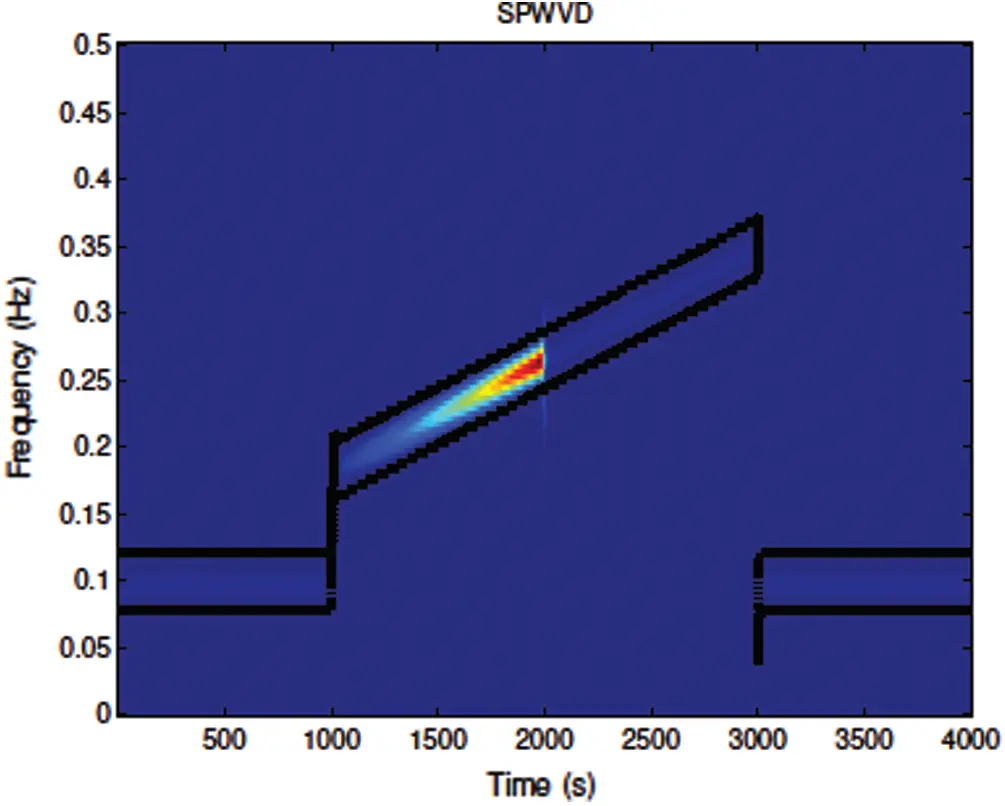Figure 1: The smoothed pseudo Wigner–Ville representation of the simulated signal, with the gabarit in black

We define the gabarit function as a function which is equal to one around the frequency band of respiration and zero elsewhere, mathematically, it can be considered as the Indicator function which is equal to one around the respiratory frequency and zero elsewhere. The gabarit plays a key role in filtering, the representation of the obtained signal will be multiplied by the gabarit function in the time frequency domain for spectrogram, SPWVD and their reassignments. After the filtered signal obtained at the frequency band of respiration, will be studied to compare which representation is better, and contains more power. In this simulated signal, we consider that the respiratory signal is concentrated around the frequencies of the signal in each part, the aim of this simulation is to show how the gabarit is well adapted to our simulated modulation .

In Fig. 2, after representing in the time frequency domain with spectrogram, SPWVD and both their reassignments  for a simulated signal, the result obtained will be multiplied by the GABresp and after that we integrate over the frequencies weighting by the number of the points used in the gabarit function. The obtained result, is quadratic in the time domain, to compare it with the original signal, we take the root square of this quantity, and we compare with the envelope of the magnitude of the starting signal as shown in Fig. 3.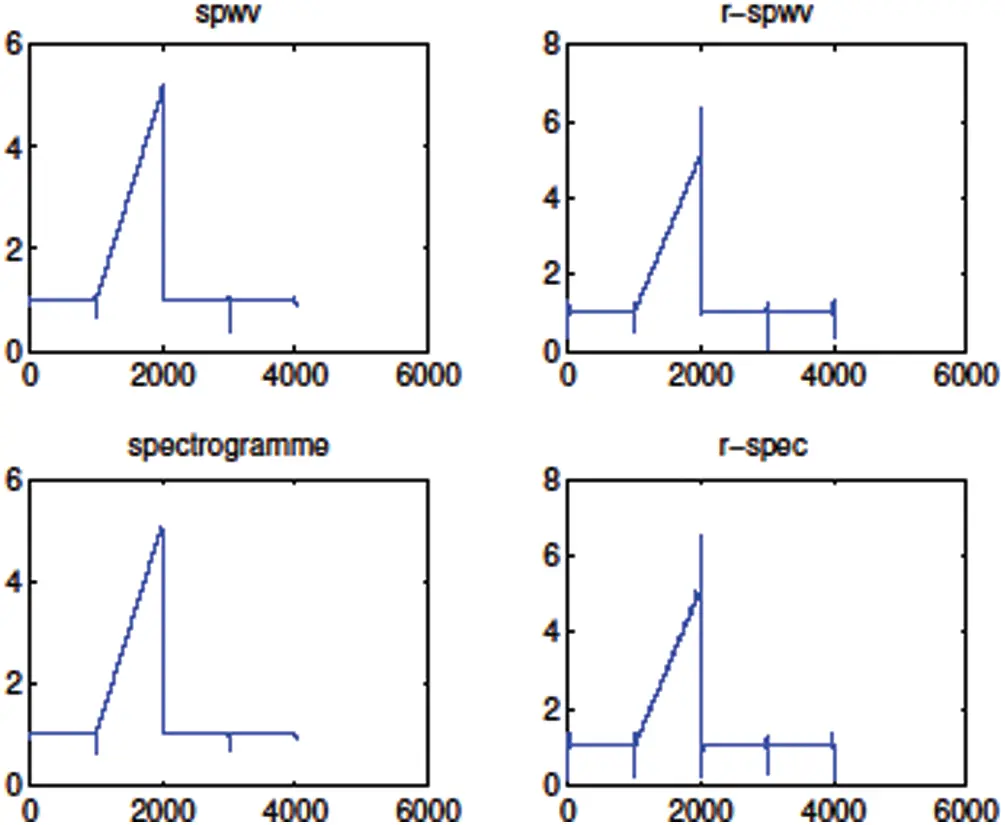Figure 2: The envelope of the magnitude of the filtered signal obtained after time frequency representation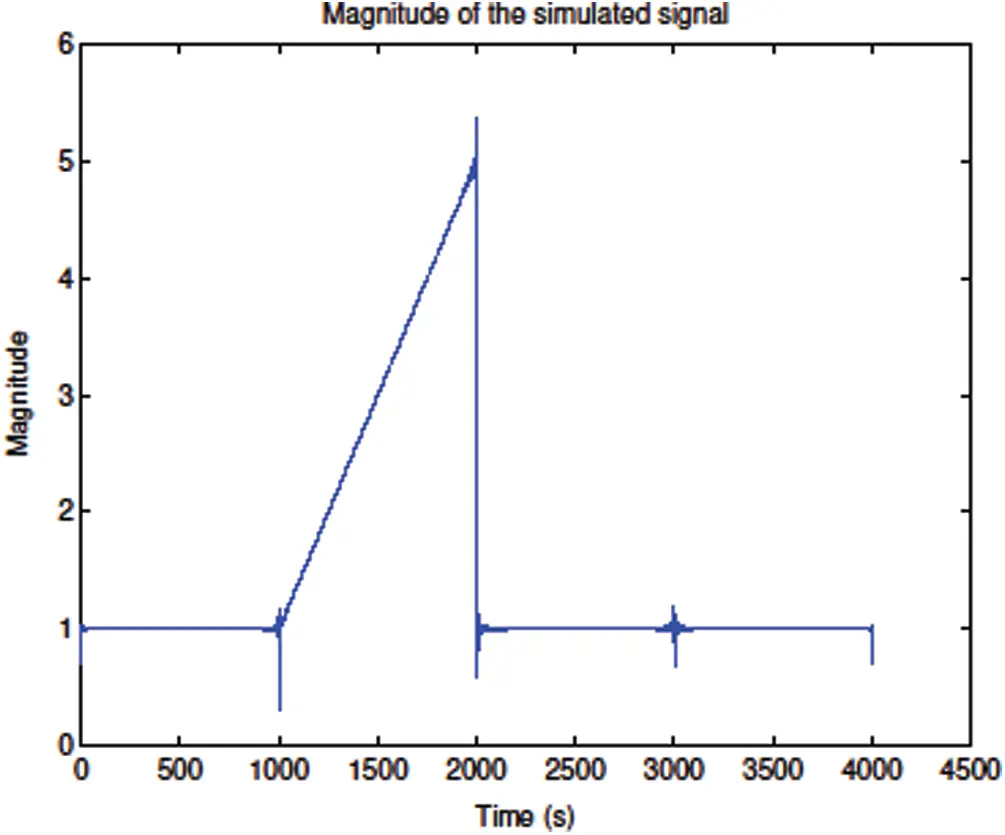Figure 3: Envelope of the magnitude of the simulated signal

5.2 Real Signals During Exercise Stress Testing

In our study, we need the instants tk, from these beat occurrences, first we obtain the instantaneous heart rate, then we get the time varying mean heart rate from the instantaneous heart rate, using a high-pass filtering, then we obtain the heart rate variability by subtracting the time varying mean heart rate from the instantaneous heart rate. Finally, the obtained HRV will be corrected by the time varying mean heart rate, and we obtain the modulating signal using the TVIPFM model.

The technique proposed in this paper is the time varying signal filtering using the gabarit function at the time frequency domain around the respiration.

The SPWVD signal X(t,υ) of the original signal x(t) is after integrated respect to the frequency υ, for spectrogram and the SPWVD and their reassignments, we expect to have the instantaneous power |x(t)|2, and

X(t,υ)dυ=h(s2)h(s2)x(t+s2)x(ts2)ei2πυsdsdυ=|x(t)|2(15)

Fig. 4. shows the time frequency representation of the HRV of a real signal using the SPWVD representation with the gabarit function (in black) around the recorded frequency band of respiration. The high correlation between the HRV obtained from the TVIPFM model and the respiration is obviously displayed.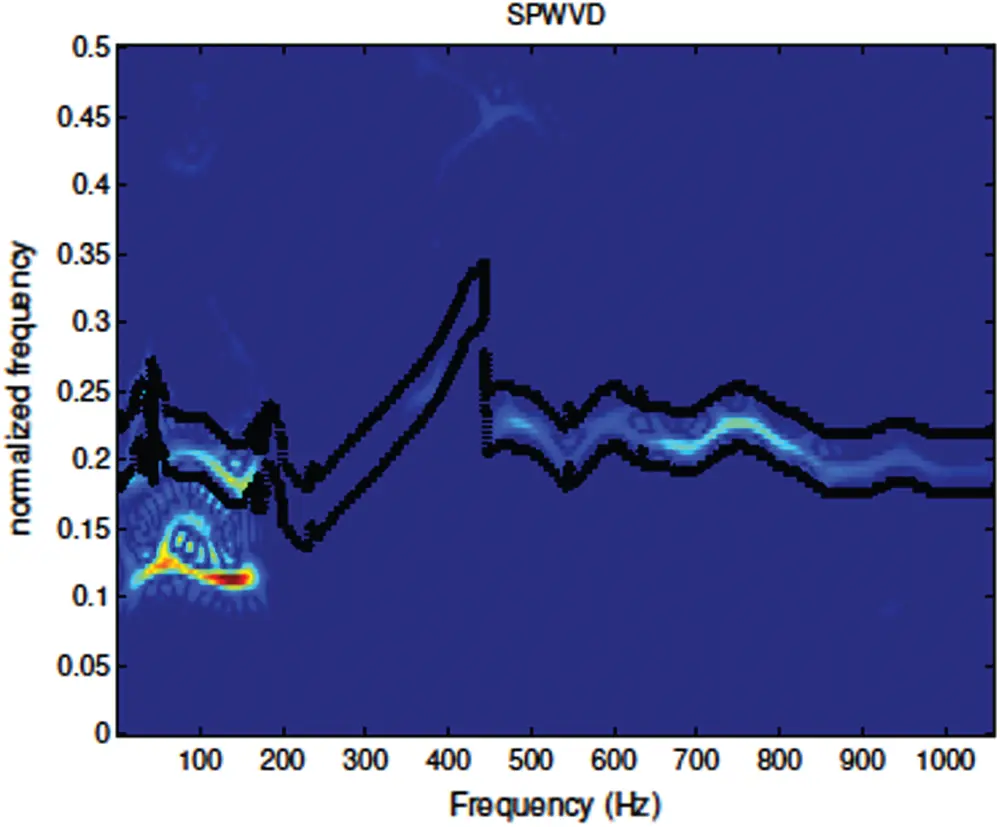Figure 4: The smoothed pseudo Wigner–Ville representation of a real signal with gabarit function

So, we obtain the magnitude of the signal x(t) after the time varying filtering, that’s why we apply the root square on this quantity to retrieve the magnitude of the real signal.

In Fig. 5, we represent the envelope of the magnitude of the modulating signal with different time frequency representations like SPWVD, spectrogram and their reassignments. Results obtained prove the importance of our time varying filtering technique to retrieve the modulation from the heart rate variability due to the respiratory sinus arrhythmia. The use of a time varying model with a time varying filtering around the respiration, gives us the best envelope using the spectrogram method.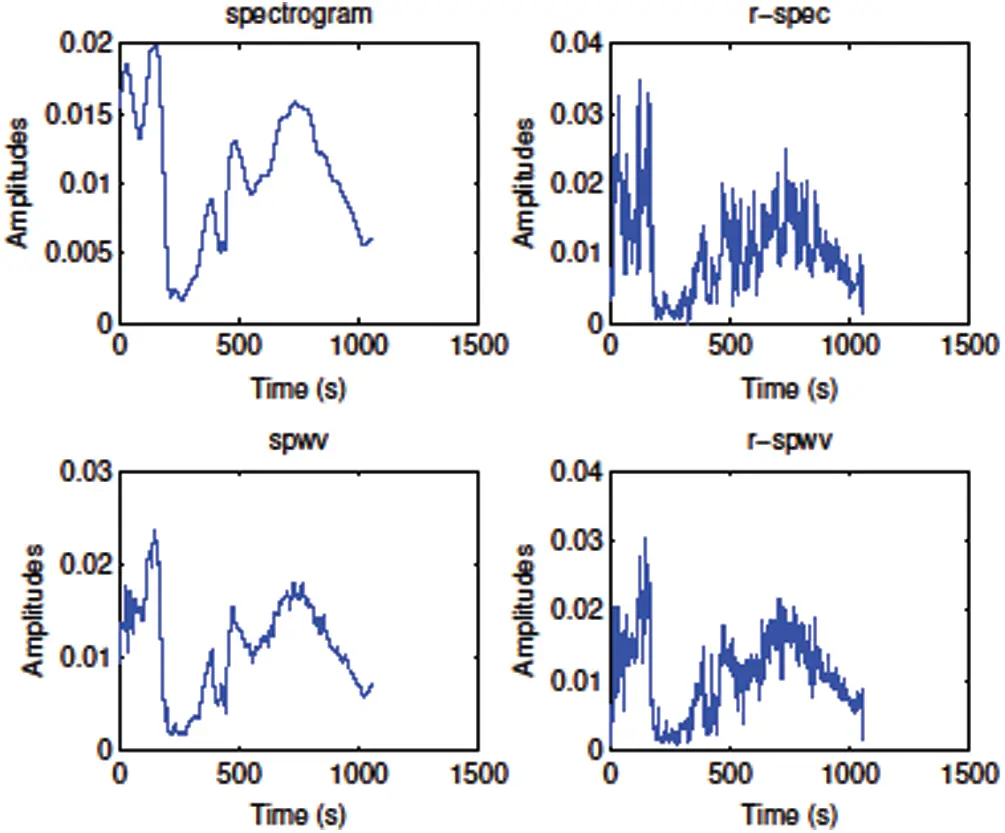Figure 5: Magnitude of the filtered real signal

In Fig. 6, we show that the correlation between the HRV and the respiration using different kinds of time frequency representations as spectrogram, SPWVD and their reassignments.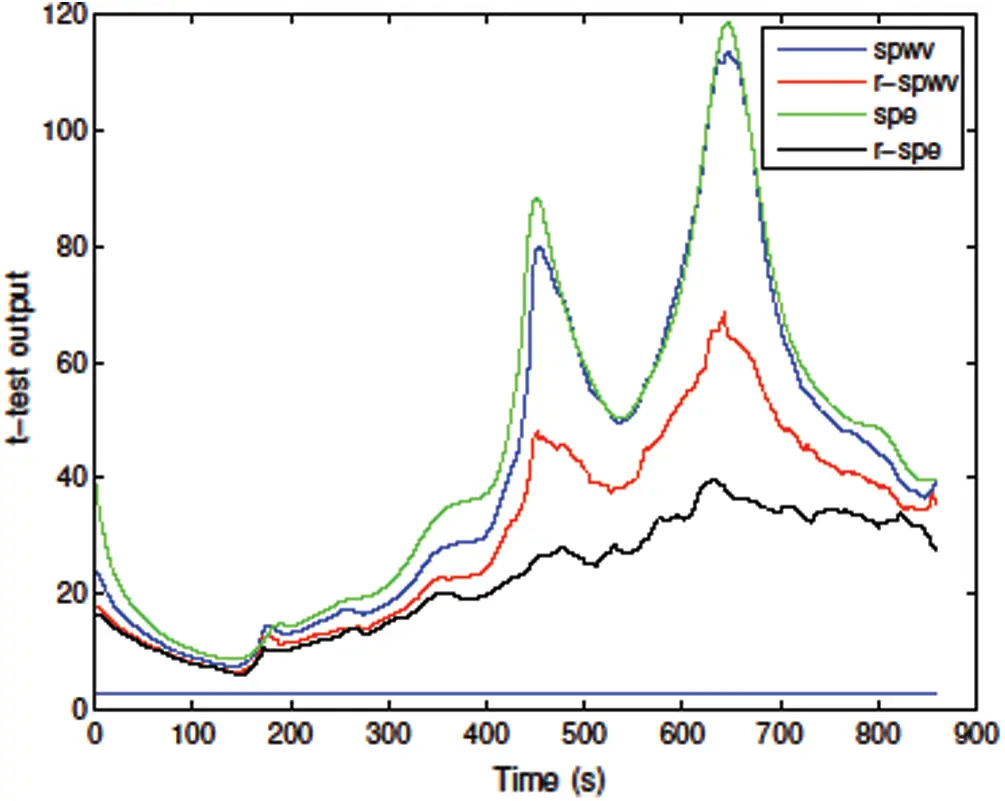Figure 6: Student t-test with significance p<0.01

There is a high correlation between the respiration and the heart rate variability, with significance p<0.01. In this figure, for each time frequency representation, the instantaneous power at the frequency band of respiration and elsewhere are computed. We obtain two sets of data. We use a t-test with a window of 200 samples. The curves spwv, r-spwv, spe and r-spe plotted in Fig. 6 represent the result of the t-test, the horizontal straight line represents the threshold of the significance level. The presence of the instantaneous power in the respiratory frequency band is always shown p<0.01 with each time frequency representation. Therefore, we can use our technique of filtering to retrieve the envelopes of the magnitude of the modulating signal corresponding to the ANS modulation. The spectrogram representation, shows the most significant correlation between the modulating signal and the respiration.

The correlation modeled between the respiration and the HRV plays a key role to understand the control of the ANS on the heart. A recent work  studied the link between respiration and HRV during yoga breathing practice. After six months of yoga, HRV showed improvement towards the parasympathetic domain. This study was performed in the time and frequency domains. Modeling the HRV coupled with the respiration has several applications. One current study  was applications was about tracking the cardiorespiratory load of firefighters reflecting the respiratory metabolism state to ensure their safety. This study needs to take into account a respiration during the intense physical exercise. The reader may refer to , where the HRV is positively linked with compassion. The study HRV associated with soothing emotions.

6  Conclusion

In this paper, we used the TVIPFM to obtain the modulating signal of the heart rate variability filtered with respect to the respiration frequency. This filtering is assumed in time frequency domain, using different representations like spectrogram, the smoothed pseudo Wigner–Ville distribution and their reassignments. Differences between magnitudes of instantaneous power in all of the representations are due to the reassignment principle moving power dispersed, to reassign it to the nearest point. After that, we used a statistical test to explain our choice to filtrate around respiration, so we involve the student t-test to compare the instantaneous power in the respiratory frequency band and elsewhere using the gabarit function. The significance of the t-test is set at p<0.01 showing that all time frequency representations used prove the existence of the power in the respiratory frequency band.

HRV has been also studied with Kalman smoothed method to estimate time varying characteristics of HRV. The proposed method, supposes that HRV is obtained by auto regressive model. Using a Kalman smoothed algorithm, parameters are estimated. In this method, the time varying spectrum is a continuous function of frequency, so we can evaluate it at any desired frequency up to the Nyquist frequency fs2. The advantage of the Kalman smoother algorithm method is that the spectrum is separable.

However, one of the limits of this method is that the characteristics of the Kalman smoother spectrum depends strongly on the order of the auto regressive model. For example, if the used model is with a higher order, it can lead to some interference in the spectrum. Studying HRV during exercise imposes the use of a non-stationary mean time varying period. So, we studied the HRV using our TVIPFM model, also we used the method of time varying filtering. Finally, with this study, we satisfy the increasing exercise conditions.

Funding Statement: This work was supported by College of Engineering and Technology, the American University of the Middle East, Kuwait. Homepage: https://www.aum.edu.kw.

Conflicts of Interest: The authors declare that they have no conflicts of interest to report regarding the present study.

## References

1. L. Bernardi, B. Bianchini, G. Spadacini, S. Leuzzi, F. Valle et al., “Demonstrable cardiac reinnervation after human heart transplantation by carotid baroreflex modulation of RR interval,” Circulation, vol. 92, no. 10, pp. 2895–2903, 1995.
2. R. Bailón, G. Laouini, C. Grao, M. Orini, P. Laguna et al., “The integral pulse frequency modulation model with time-varying threshold: Application to heart rate variability analysis during exercise stress testing,” IEEE Transactions on Biomedical Engineering, vol. 58, no. 3, pp. 642–652, 2010.
3. A. L. Goldberger, “Is the normal heartbeat chaotic or homeostatic?,” Physiology, vol. 6, no. 2, pp. 87–91, 1991.
4. F. Beckers, B. Verheyden and A. E. Aubert, “Aging and nonlinear heart rate control in a healthy population,” American Journal of Physiology-Heart and Circulatory Physiology, vol. 290, no. 6, pp. H2560–H2570, 2006.
5. D. E. Vaillancourt and K. M. Newell, “Changing complexity in human behavior and physiology through aging and disease,” Neurobiology of Aging, vol. 23, no. 1, pp. 1–11, 2002.
6. E. G. Caiani, A. Martin-Yebra, F. Landreani, J. Bolea, P. Laguna et al., “Weightlessness and cardiac rhythm disorders: Current knowledge from space flight and bed-rest studies,” Frontiers in Astronomy and Space Sciences, vol. 3, pp. 27, 201
7. P. K. Stein, P. P. Domitrovich, N. Hui, P. Rautaharju and J. Gottdiener, “Sometimes higher heart rate variability is not better heart rate variability: Results of graphical and nonlinear analyses,” Journal of Cardiovascular Electrophysiology, vol. 16, no. 9, pp. 954–959, 2005.
8. R. D. Lane, E. M. Reiman, G. L. Ahern and J. F. Thayer, “21. Activity in medial prefrontal cortex correlates with vagal component of heart rate variability during emotion,” Brain and Cognition, vol. 47, no. 1–2, pp. 97–100, 2001.
9. E. J. Bayly, “Spectral analysis of pulse frequency modulation in the nervous systems,” IEEE Transactions on Biomedical Engineering, vol. 4, no. 4, pp. 257–265, 1968.
10. R. Bailón, L. Mainardi, M. Orini, L. Sörnmo and P. Laguna, “Analysis of heart rate variability during exercise stress testing using respiratory information,” Biomedical Signal Processing and Control, vol. 5, no. 4, pp. 299–310, 20
11. S. Michael, K. S. Graham and G. M. Davis, “Cardiac autonomic responses during exercise and post-exercise recovery using heart rate variability and systolic time intervals-a review,” Frontiers in Physiology, vol. 8, pp. 301, 2017.
12. J. Mateo and P. Laguna, “Improved heart rate variability signal analysis from the beat occurrence times according to the IPFM model,” IEEE Transactions on Biomedical Engineering, vol. 47, no. 8, pp. 985–996, 2000.
13. J. Mateo and P. Laguna, “Analysis of heart rate variability in the presence of ectopic beats using the heart timing signal,” IEEE Transactions on Biomedical Engineering, vol. 50, no. 3, pp. 334–343, 2003.
14. G. Laouini, A. Cabasson, G. Blain, P. Bonizzi, O. Meste et al., “Evidence of the influence of respiration on the heart rate variability after human heart transplantation: Role of observation model,” in 2009 36th Annual Computers in Cardiology Conf., Park City, UT, USA, pp. 409–412, 2009.
15. O. Meste, G. Blain and S. Bermon, “Some considerations on the IPFM model for the heart rate variability analysis,” in Computers in Cardiology, Thessaloniki, Greece, pp. 709–712, 2003.
16. O. Meste, B. Khaddoumi, G. Blain and S. Bermon, “Time-varying analysis methods and models for the respiratory and cardiac system coupling in graded exercise,” IEEE Transactions on Biomedical Engineering, vol. 52, no. 11, pp. 1921–1930, 2005.
17. G. Blain, O. Meste, T. Bouchard and S. Bermon, “Assessment of ventilatory thresholds during graded and maximal exercise test using time varying analysis of respiratory sinus arrhythmia,” British Journal of Sports Medicine, vol. 39, no. 7, pp. 448–452, 2005.
18. M. Qaraqe, M. Ismail and E. Serpedin, “Combined matching pursuit and Wigner–Ville distribution analysis for the discrimination of ictal heart rate variability,” in 2016 24th European Signal Processing Conf., Budapest, Hungary, pp. 2045–2049, 2016.
19. S. Pola, A. Macerata, M. Emdin and C. Marchesi, “Estimation of the power spectral density in nonstationary cardiovascular time series: Assessing the role of the time-frequency representations (TFR),” IEEE Transactions on Biomedical Engineering, vol. 43, no. 1, pp. 46, 1996.
20. M. Hansson and P. Jönsson, “Estimation of HRV spectrogram using multiple window methods focusing on the high frequency power,” Medical Engineering & Physics, vol. 28, no. 8, pp. 749–761, 2006.
21. A. K. Barros and N. Ohnishi, “Heart instantaneous frequency (HIFAn alternative approach to extract heart rate variability,” IEEE Transactions on Biomedical Engineering, vol. 48, no. 8, pp. 850–855, 2001.
22. W. Martin and P. Flandrin, “Wigner–Ville spectral analysis of nonstationary processes,” IEEE Transactions on Acoustics, Speech, and Signal Processing, vol. 33, no. 6, pp. 1461–1470, 1985.
23. O. Rompelman, J. B. I. M. Snijders and C. J. Van Spronsen, “The measurement of heart rate variability spectra with the help of a personal computer,” IEEE Transactions on Biomedical Engineering, vol. 29, no. 7, pp. 503–510, 1982.
24. F. Auger and P. Flandrin, “Improving the readability of time-frequency and time-scale representations by the reassignment method,” IEEE Transactions on Signal Processing, vol. 43, no. 5, pp. 1068–1089, 1995.
25. R. Bailon, P. Laguna, L. Mainardi and L. Sornmo, “Analysis of heart rate variability using time-varying frequency bands based on respiratory frequency,” in 2007 29th Annual Int. Conf. of the IEEE Engineering in Medicine and Biology Society, Lyon, France, pp. 6674–6677, 2007.
26. M. Kuppusam, D. Kamaldeen, R. Pitani, J. Amaldas, P. Ramasamy et al., “Effects of yoga breathing practice on heart rate variability in healthy adolescents: a randomized controlled trial,” Integrative Medicine Research, vol. 9, no. 1, pp. 28–32, 2020.
27. Y. Ito, Y. Oka and Y. Kuriyam, “Applicability of the reflection index of respiration based on heart rate variability analysis to firefighting activity,” Fire Science and Technology, vol. 39, no. 1, pp. 1–5, 2020.
28. M. Di Bello, L. Carnevali, N. Petrocchi, J. F. Thayer, P. Gilbert et al., “The compassionate vagus: A meta-analysis on the connection between compassion and heart rate variability,” Neuroscience & Biobehavioral Reviews, vol. 116, no. 1, pp. 21–30, 2020.This work is licensed under a Creative Commons Attribution 4.0 International License, which permits unrestricted use, distribution, and reproduction in any medium, provided the original work is properly cited.